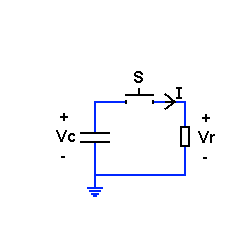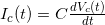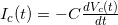Here, as promised, is the solution to the RC circuit puzzle I posted earlier this week. Let's look at the circuit again:The problem with my reasoning was the direction of current in the capacitor. I've quietly assumed that:But this is wrong for the circuit above. Why? Because we must obey the voltage & current directions we've chosen. In passive elements, the positive current flows from the higher voltage to the lower voltage, meaning that in our circuit:This small minus sign makes all the difference, and now the solution will be correct. Physically, the intuition is that the current here flows from a discharging capacitor, hence it's "against" the voltage direction. Had it been a capacitor-charging circuit, there would be no confusion.